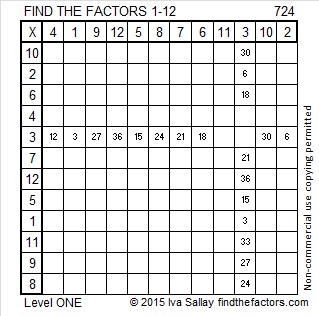# 724 and Level 1

• 724 is a composite number.
• Prime factorization: 724 = 2 x 2 x 181, which can be written 724 = (2^2) x 181
• The exponents in the prime factorization are 2 and 1. Adding one to each and multiplying we get (2 + 1)(1 + 1) = 3 x 2  = 6. Therefore 724 has exactly 6 factors.
• Factors of 724: 1, 2, 4, 181, 362, 724
• Factor pairs: 724 = 1 x 724, 2 x 362, or 4 x 181
• Taking the factor pair with the largest square number factor, we get √724 = (√4)(√181) = 2√181 ≈ 26.907248.Solving this Level 1 puzzle will be even easier:Print the puzzles or type the solution on this excel file: 12 Factors 2015-12-28

————————————————————

Here is a little more about the number 724:

87 + 88 + 89 + 90 + 91 + 92 + 93 + 94 = 724; that’s 8 consecutive numbers.

724 is the sum of the 6 prime numbers from 107 to 137.

724 is also the sum of the 4 primes from 173 to 191.

18² + 20² = 724.

Because 181 is one of its factors, 724 is the hypotenuse of the Pythagorean triple 76-720-724. What 1-digit number is the greatest common factor of those three numbers?

724 is palindrome 202 in BASE 19; note that 2(19²) + 0(19) + 2(1) = 724.

—————————————————————This site uses Akismet to reduce spam. Learn how your comment data is processed.